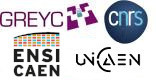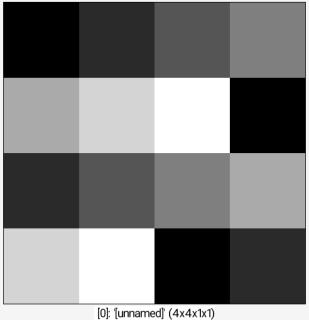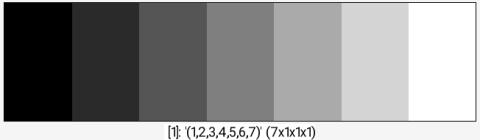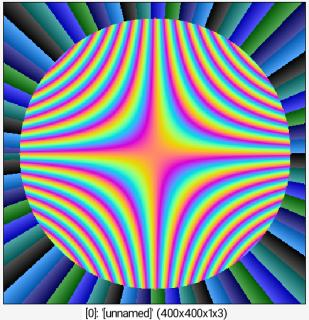G'MIC - GREYC's Magic for Image Computing: A Full-Featured Open-Source Framework for Image Processing# A Full-Featured Open-Source Framework for Image Processing

## Latest stable version: 3.3.0        Current pre-release: 3.3.1 (2023/09/16)

Reference

 Table of Contents  ▸  List of Commands  ▸  Values Manipulation  ▸  fill ◀  equalize    |    index  ▶

# fill

Built-in command

## Arguments:

• value1,_value2,...    or
• [image]    or
• 'formula'

## Description:

Fill selected images with values read from the specified value list, existing image

or mathematical expression. Single quotes may be omitted in formula.

(equivalent to shortcut command f).

This command has a .

## Examples of use:

### • Example #1

4,4 fill 1,2,3,4,5,6,7Command: 4,4 fill 1,2,3,4,5,6,7

### • Example #2

4,4 (1,2,3,4,5,6,7) fill[-2] [-1]Command: 4,4 (1,2,3,4,5,6,7) fill[-2] [-1]Command: 4,4 (1,2,3,4,5,6,7) fill[-2] [-1]

### • Example #3

400,400,1,3 fill "X=x-w/2; Y=y-h/2; R=sqrt(X^2+Y^2); a=atan2(Y,X); if(R<=180,255*abs(cos(c+200*(x/w-0.5)*(y/h-0.5))),850*(a%(0.1*(c+1))))"Command: 400,400,1,3 fill "X=x-w/2; Y=y-h/2; R=sqrt(X^2+Y^2); a=atan2(Y,X); if(R<=180,255*abs(cos(c+200*(x/w-0.5)*(y/h-0.5))),850*(a%(0.1*(c+1))))"
G'MIC - GREYC's Magic for Image Computing: A Full-Featured Open-Source Framework for Image Processing